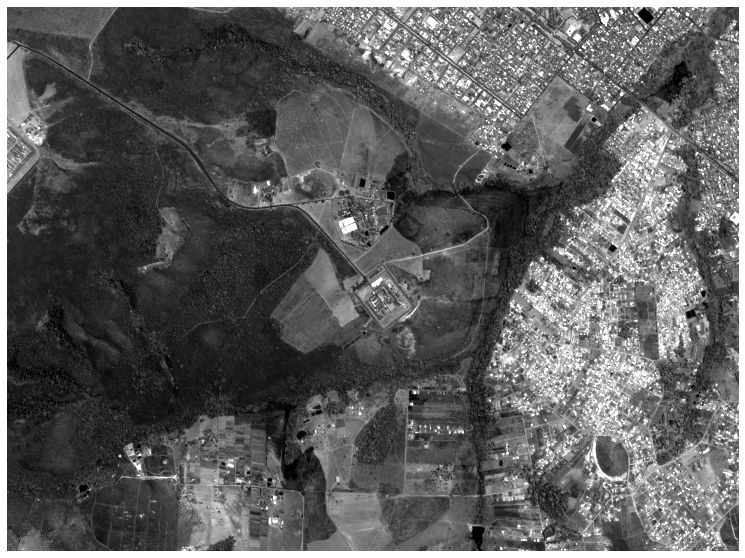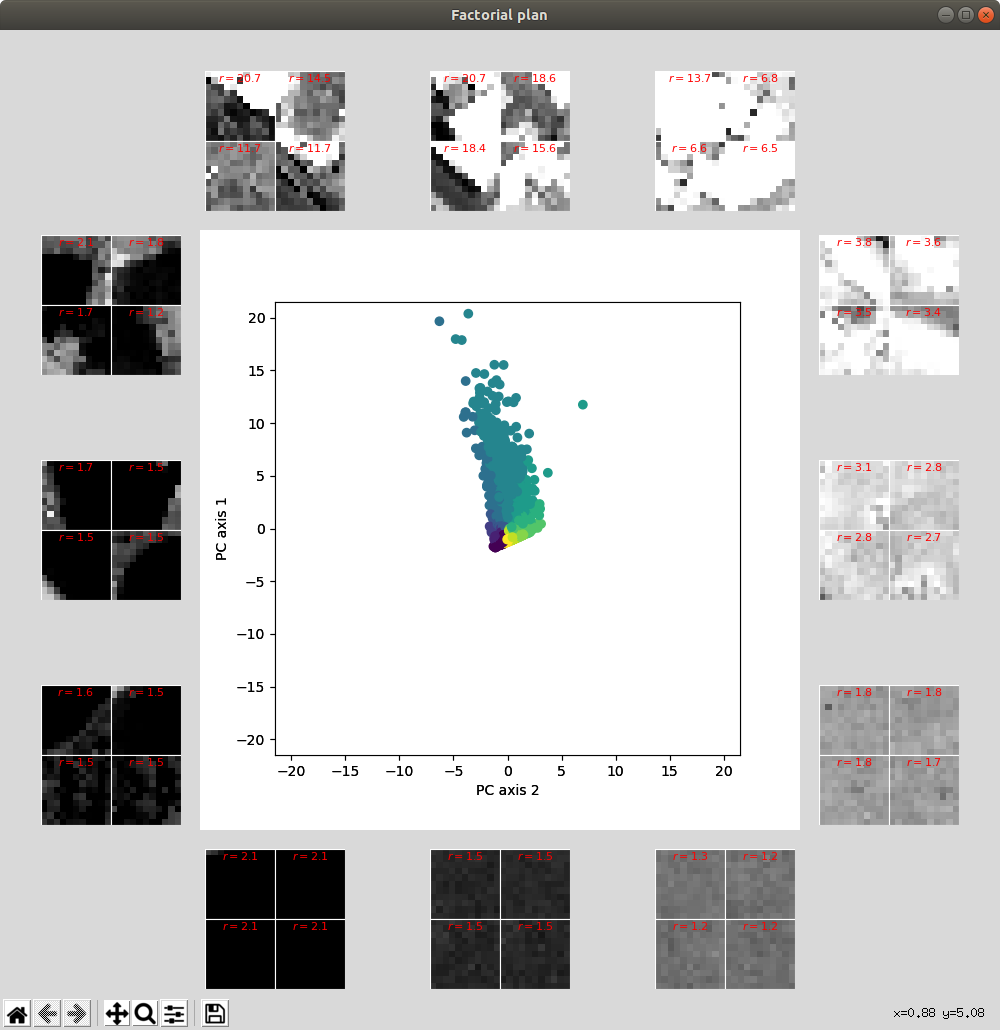# Fourier Transform Textural Ordination in Python¶

## 1. Introduction¶

The fototex package implements the FOTO algorithm described in Textural ordination based on Fourier spectral decomposition: a method to analyze and compare landscape patterns (Pierre Couteron, Nicolas Barbier and Denis Gautier, 2006), which allows texture characterization and comparison. Though this algorithm has already been implemented in MATLAB and R, fototex enriches this collection by both implementing new features and dramatically enhancing performance.

## 2. Basic usage¶

The main module of fototex is foto, in which various classes might be used and combined to structure your own FOTO algorithm, suited to your own needs. Here is the list of the main available classes at the moment:

class FotoBase
class Batch(FotoBase)
class Sector(FotoBase)
class Foto(FotoBase)
class FotoBatch(Batch)
class FotoSector(Foto, Sector)
class FotoSectorBatch(FotoBatch, Sector)

• FotoBase: base class describing the core of the FOTO algorithm
• Batch: base subclass implementing image batch supply
• Sector: base subclass implementing radial spectra anisotropy calculation
• Foto: subclass implementing the typical FOTO algorithm, i.e. the isotropic FOTO algorithm on one given image

By using inheritance and multiple inheritance, you can then combine the different features between them. Typically, at the moment, those subclasses are the following:

• FotoBatch: inherits from Batch and allows the FOTO algorithm to be implemented from multiple image batches
• FotoSector: inherits from both Foto and Sector classes, and implements anisotropic FOTO
• FotoSectorBatch: inherits from both FotoBatch and Sectorclasses, and implements anisotropic FOTO from multiple image batches

### 2.1 Main features¶

#### 2.1.1 Sliding window method¶

The FotoBase gives you 2 methods for sliding window over an image or image batches. The first is the block method (method="block") that computes r-spectra for each window in the image block by block. The second is the moving method (method="moving_window") that computes r-spectra for a window sliding from West to East and North to South. This method requires far more CPU resources. In that case, the total number of windows will be the size of the input image. For example, an image that would require 150k block 11x11 windows would require about 20M windows in moving mode.

#### 2.1.2 Isotropic vs. anisotropic R-spectra¶

Isotropic r-spectra does not look at the direction but rather averages the spectra over the entire azimuth (i.e. from 0° to 360°). Conversely, anisotropic calculation takes care of the direction, that is the angular sector for which the spectra must be averaged. In the main classes of the fototex package, everything that is not Sector based is isotropic, and everything that is Sector based is anisotropic. In the anisotropic case, the maximum number of sectors should somehow be given by the square pixel-based geometry of a window: 8 as the number of pixels surrounding the window center. However, it is possible to increase this number by considering nearest neighboring for specific radius values. In other words, it is not possible to get 12 bin sectors for the first 8 surrouding pixels, but the 4 "in-between" sectors that are not digitally represented might be assessed from the nearest available values.

#### 2.1.3 Single image against set of image batches¶

The basic FOTO algorithm takes one single image as input. In order to take into account different textures in the training process from different places and images, you can use the Batch subclass. Everything that is Batch implements FOTO algorithms from multiple image batches, and everything without implements the normal algorithm.

#### 2.1.4 In memory against H5 hard storage¶

Whether your machine has a lot of memory of the image size is not too large, the FotoBase subclasses and processes might be run in memory (in_memory=True). Conversely, if for any reason you are out of memory, there is still the possibility to run the algorithm using H5 hard temporary storage. In this case, be warned that io reading and writing will increase the CPU time. You may adjust the size of the chunks to be read from and written to the H5 temporary file by setting the data_chunk_size argument.

#### 2.1.5 Multiprocessing¶

Everything in the fototex package is parallelized (except io reading/writing). The number of processes is available through nb_processes in the different FotoBase class and subclass methods. By default, this number is set to the maximum available number of CPU on your machine (nb_processes=mp.cpu_count()). In case you do not want to blow your computer off, you may want to reduce this number.

### 2.4 The FotoBase class and subclasses¶

#### 2.4.1 The Foto class¶

This class implements the main FOTO algorithm, i.e. computes isotropic r-spectra and applies dimensionality reduction through Principal Component Analysis.

Constructor:

Foto(image, band=None, method="block", in_memory=True, data_chunk_size=50000)


Required parameters:

• image: (str) path to a valid GeoTIFF raster/image

Optional parameters:

• band: (int) band number if multiband raster
• method: (str) valid window method among {"block", "moving_window"}
• in_memory: (bool) either implement FOTO algorithm (r-spectra and PCA) in memory or use HDF5 file storage on the fly
• data_chunk_size: (int) if HDF5 storage is used, the number of data per chunk for reading/writing to file

#### 2.4.2 The FotoSector class¶

The FotoSector class implements the calculation of anisotropic r-spectra, that is averaged r-spectra for given angular sectors. It is possible to give the desired orientation to those r-spectra, by setting the direction of the starting sector. By default, it is set to 0°, i.e. North (start_sector=0).

Constructor:

FotoSector(image, nb_sectors=6, start_sector=0, band=None, method="block", in_memory=True,
data_chunk_size=50000)


Required parameters:

• image: (str) path to a valid GeoTIFF raster/image

Optional parameters:

• nb_sectors: (int) number of sectors for which r-spectra must be computed (default: 6, max: Sector.max_nb_sectors = fototex.MAX_NB_SECTORS)
• start_sector: (float, int) starting sector direction (default: 0°, i.e. North)
• band: (int) band number if multiband raster
• method: (str) valid window method among {"block", "moving_window"}
• in_memory: (bool) either implement FOTO algorithm (r-spectra and PCA) in memory or use HDF5 file storage on the fly
• data_chunk_size: (int) if HDF5 storage is used, the number of data per chunk for reading/writing to file

### 2.5 Main methods¶

#### 2.5.1 The run method¶

The run method typically consists in computing r-spectra for each window in either the image or set of image batches and then applying dimensionality reduction through a Principal Component Analysis (PCA).

Usage:

Foto.run(window_size, nb_sampled_frequencies=None, standardized=True, normalized=False,
keep_dc_component=False, at_random=False, batch_size=None, max_iter=1000,
nb_processes=mp.cpu_count())


Required parameters:

• window_size: (int) size of the sliding window that will be passed throughout the image

Optional parameters:

• nb_sampled_frequencies: (int) number of frequencies sampled within window. If None, is inferred
• standardized: (bool) if True, standardize (z-score) r-spectrum data before PCA
• normalized: (bool) if True, divide power spectrum density by window's variance
• keep_dc_component: (bool) if True, keep DC component (frequency 0) when computing r-spectra
• at_random: (bool) when HDF5 storage is active (in_memory == False), if True use random batches for incremental PCA, otherwise use the whole dataset
• batch_size: (int) when using incremental PCA at random, define the size of the batch
• max_iter: (int) when using incremental PCA at random, maximum number of iterations before stopping the process
• nb_processes: (int) number of processes for multiprocessing (by default, all CPU are used)

#### 2.5.2 The save methods (save_rgb, save_data)¶

Typically, there are 2 main save methods that allow to save either the RGB mapping of reduced r-spectra or the different data generated by the FOTO algorithm into some H5 file.

Usage:

Foto.save_rgb()


Save reduced r-spectra to RGB map (as a GeoTiff fill by default)

Foto.save_data()


Save FOTO algorithm data (r-spectra, reduced r-spectra and eigen vectors, as well as attributes such as window size and sliding method) as datasets into one unique H5 file. You may also use save_eigen_vectors, save_r_spectra and r_reduced_r_spectra individually.

#### 2.5.3 The plot method¶

This method allows for plotting of the reduced r-spectra in the factorial plan $(r, \theta)$ defined by PC axis 1 and PC axis 2. For each quadrant $\theta$, windows corresponding to reduced r-spectra values are selected (either at random or based on the maximum norm values in quadrant $\theta$). You may either run the plot method on a Foto instance that has just run or use results previously stored by implementing the h5path argument.

Usage:

Foto.plot(h5path=None, nb_points=10000, data_range=None,
main_fig_rel_size=0.6, contrast_range=(2, 98), *args, **kwargs)


Optional parameters:

• h5path: (str) Path to HDF5 file where reduced r-spectra are stored. If None, instance r-spectra are used. Useful for later plotting.
• nb_points: (int) Number of PC points to be plotted
• data_range: (list[float, float]) Data range as cumulative cut count for PC axis1 and axis2 (e.g. [2, 98])
• nb_quadrants: (int) Number of quadrants ($\theta$) the factorial plan must be divided in
• norm_method: (str) Method used to retrieve windows in each quadrant:
• 'max' retrieve window(s) with respect to maximum norm
• 'random' retrieve window(s) at random in quadrant
• nb_windows_per_side: (int) Number of windows per side (Each quadrant corresponds to a square set of windows such as 1x1, 2x2, 3x3, etc.)
• main_fig_rel_size: (float) Relative size of central figure between 0 and 1
• contrast_range: (list[float, float]) Percentile contrast range used to render windows with respect to the whole image. ex.: enhance contrast based on cumulative count cut between 2% and 98% --> [2, 98]

### 2.6 Examples¶

Import everything we need

In :
import os
from fototex.foto import Foto, FotoSector, FotoBatch, FotoSectorBatch
from fototex.foto_tools import degrees_to_cardinal
import numpy as np
from matplotlib import pyplot as plt
from skimage import io


Let's read one small sample image to see what we can do with it:

In :
image_file = os.path.join("tutorial", "pan_image_test.tif")

In :
plt.rcParams['figure.figsize'] = [15, 10]
img = plt.imshow(sample_image, cmap='gray', vmin=50, vmax=106)
plt.axis('off')

Out:
(-0.5, 2240.5, 1665.5, -0.5)#### 2.6.1 Run the isotropic FOTO algorithm on a given image¶

Build a simple Foto instance, using the block method and applying the process in memory:

In :
foto_obj = Foto(image_file, method="block", in_memory=True)


Now we can run it with a window size of 11:

In :
foto_obj.run(window_size=11, keep_dc_component=True)

Retrieve isotropic R-spectra: 100%|██████████| 31.0k/31.0k [00:02<00:00, 13.6kit/s]
Run Principal Component Analysis: 100%|██████████| 1.53k/1.53k [00:00<00:00, 94.6kit/s]


We may plot resulting reduced R-spectra in the factorial plan:

In :
foto_obj.plot()We can also save the reduced r-spectra as a RGB map:

In :
foto_obj.save_rgb()

Write RGB output image to 'tutorial/pan_image_test_method=block_wsize=11_rgb.tif': 100%|██████████| 152/152 [00:00<00:00, 6.91kit/s]


Now we can open this image, read it and look at what it looks like:

In :
rgb_image = io.imread(foto_obj.rgb_file)
#rgb_image[rgb_image==-999] = np.nan

# 2%-98% range for values in each channel
value_min = [-1.42, -1.04, -1.29]
value_max = [6.28, 1.56, 1.22]

for vmin, vmax, band in zip(value_min, value_max, range(rgb_image.shape)):
rgb_image[:,:,band] = (rgb_image[:,:,band] - vmin)/ (vmax - vmin)

In :
plt.imshow(rgb_image)
plt.axis('off')

Clipping input data to the valid range for imshow with RGB data ([0..1] for floats or [0..255] for integers).

Out:
(-0.5, 203.5, 151.5, -0.5)